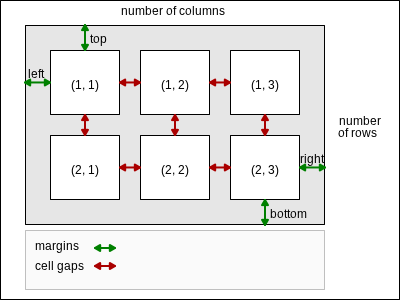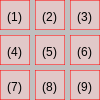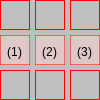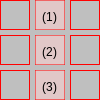# OEImageGrid¶

class OEImageGrid


This class represents OEImageGrid which is a layout manager that aligns cells into rows and columns. The cells of the grid are equal-sized and evenly spaced. See Figure: Schematic representation of an OEImageGrid object.Schematic representation of an OEImageGrid object

Note

In the image grid, each cell has the same width and height. The width and height of the cells are calculated based on the height and width of the OEImageGrid, its margins and gap set between the cells.

## Constructors¶

OEImageGrid(OEImageBase &parent, unsigned int rows, unsigned int cols)


Creates an OEImageGrid object with the specified number of rows and columns.

parent

The OEImageBase object on which the OEImageGrid object is placed.

rows

The number of rows in the OEImageGrid object.

cols

The number of columns in the OEImageGrid object.

Note

The width and the height of the OEImageGrid object are identical to the width and height of the parent OEImageBase, respectively.

## GetCell¶

OEImageBase *GetCell(unsigned int r, unsigned int c)


Returns the OEImageBase pointer for the cell positioned in the $$r^{th}$$ row and $$c^{th}$$ columns.

r

This value has to be in the range from 1 to OEImageGrid.NumRows().

c

This value has to be in the range from 1 to OEImageGrid.NumCols().

## GetCellGap¶

double GetCellGap() const


Returns the space between the cells of the OEImageGrid object.

## GetCellHeight¶

double GetCellHeight() const


Returns the height of the cells of the OEImageGrid object.

## GetCellWidth¶

double GetCellWidth() const


Returns the width of the cells of the OEImageGrid object.

## GetCells¶

OESystem::OEIterBase<OEImageBase> *GetCells(unsigned int row=0,
unsigned int col=0)


Returns an iterator over the cells of the OEImageGrid object. The cells are returned from left to right and top to bottom order.

row

This value has to be in the range from 0 to OEImageGrid.NumRows().

col

This value has to be in the range from 0 to OEImageGrid.NumCols().

foreach (OEImageBase cell in grid.GetCells())Example of accessing all cells of the grid.

(The numbers inside the cells represent the order in which the cells are returned)

If the col number is zero then OEImageGrid.GetCells returns an iterator over the cells of the specified row.

uint row = 2;
foreach (OEImageBase cell in grid.GetCells(row, 0))Example of accessing a specific row of the grid.

(The numbers inside the cells represent the order in which the cells are returned)

If the row number is zero then OEImageGrid.GetCells returns an iterator over the cells of the specified column.

uint col = 2;
foreach (OEImageBase cell in grid.GetCells(0, col))Example of accessing a specific column of the grid.

(The numbers inside the cells represent the order in which the cells are returned)

## GetMargin¶

double GetMargin(unsigned int margin) const


Returns the size of a specific margin of the OEImageGrid object.

margin

This value has to be from the OEMargin namespace.

## NumCols¶

unsigned int NumCols() const


Returns the number of columns of the OEImageGrid object.

## NumRows¶

unsigned int NumRows() const


Returns the number of rows of the OEImageGrid object.

## SetCellGap¶

bool SetCellGap(double size)


Sets the space between the cells of the OEImageGrid object to the given size.

size

This value has to be a positive number.

## SetMargin¶

bool SetMargin(unsigned int margin, double size)


Sets the size of a specific margin of the OEImageGrid object.

margin

This value has to be from the OEMargin namespace.

size

This value has to be a positive number.

bool SetMargins(double size)